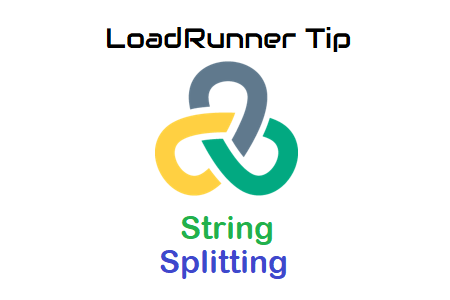In one of the scenario, I was getting ‘Address’ as a dynamic value which is a combination of the following Parameters.

• Street Name
• House Number
• Zip Code
• City
• Country

I have captured the ‘Address’ via correlation in my script. However, there are subsequent requests in which I need to pass Street Name, House Number, Zip Code, City and Country separately.

I was getting the ‘Address’ string as below.

Here ‘ ‘(space) is a token to separate each string.

To get Street Name, House Number, Zip Code, City and Country separately I have written below code.

``````Action()
{

char a,b,c,d,e;
char *temp;

temp=(char *)strtok(lr_eval_string("{c_Street}")," ");
lr_save_string(temp,"c_Street");
a=lr_output_message("%s",lr_eval_string("{c_Street}"));
lr_output_message("the string a = %s",lr_eval_string("{c_Street}"));

temp=(char *) strtok(NULL," ");
lr_save_string(temp,"c_housnumber");
b=lr_output_message("%s",lr_eval_string("{c_housnumber}"));
lr_output_message("the string b = %s",lr_eval_string("{c_housnumber}"));

temp=(char *) strtok(NULL," ");
lr_save_string(temp,"c_Zipcode");
c=lr_output_message("%s",lr_eval_string("{c_Zipcode}"));
lr_output_message("the string c = %s",lr_eval_string("{c_Zipcode}"));

temp=(char *) strtok(NULL," ");
lr_save_string(temp,"c_city");
d=lr_output_message("%s",lr_eval_string("{c_city}"));
lr_output_message("the string d = %s",lr_eval_string("{c_city}"));

temp=(char *) strtok(NULL," ");
lr_save_string(temp,"c_country");
e=lr_output_message("%s",lr_eval_string("{c_country}"));
lr_output_message("the string e = %s",lr_eval_string("{c_country}"));

return 0;
}``````